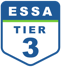Pranalika Mahanta

All Posts

It is a known fact that students sometimes struggle to cope up with the pressure of an extensive school syllabus, assignments, homework and more. Especially when it comes to math, students find it particularly challenging to balance class lessons, assignments, tests, and homework together. Therefore, it is important that teachers create a healthy classroom environment that not only focuses on solving questions, learning equations, finishing assignments but also gives students the liberty to enjoy learning through math games, brainteasers, and puzzles.

To take the pressure off students, teachers can frequently come up with fun brain teasers and math puzzles that create a fun learning environment in the class and re-energize the students. Another amazing benefit of solving math puzzles is that it encourages critical thinking in children and improves their logical reasoning skills. Therefore, it is recommended that teachers introduce fun brain teasers and puzzles along with ongoing math lessons.

Here’s a list of fun math puzzles to engage your students in math class.

## 1. Coin Triangle

This is a fun brain teaser. The motive of this puzzle is to simply turn the triangle upside down moving only 3 coins. Ask your students to solve this puzzle and to make it more fun and challenging you can set a time limit for the puzzle.

This might look easy, but this puzzle will take up quite some time. Click here to see if you got the correct answer.

## 2. Matchstick Puzzles

Matchstick puzzles are a head-scratcher and particularly fun to solve. You can use these two popular matchstick puzzles in your class and see who solves it first.

The first matchstick puzzle is called ‘turn the fish’ where students need to make the fish swim in the opposite direction by moving only 3 matchsticks. The second puzzle is also somewhat alike wherein students need to convert the 3 equal square into 2 squares by moving only 3 matchsticks.

This puzzle looks easy but is quite a thinker. Check out the answers for each of the puzzles here.

Check out our blog on 5 fun math games to engage your classroom- 5 Fun Math Games To Play In Math Class!

## 3. Sudoku

Sudoku is a classic math puzzle that has been going on for generations. It is also a great way to encourage logical thinking in students and improve their problem-solving skills.

The best part is sudoku does not have to be a tricky 3X3 grid with 9 variables, if your students are new to this puzzle you can start with an easy with 2×2 grid with 4 variables and then increase the difficulty level gradually.

Check out the answer to all three sudoku puzzles here

## 4. Prodigy

For a more holistic approach to math, you can also try Prodigy. Prodigy is a free game-based math platform for students that has numerous math challenges and puzzles for grades 1-8 and helps them in solving curriculum-aligned math problems while battling monsters, earning badges, going on quests and more.

This math platform is specifically built to engage students so that they are able to solve math problems and learn about new topics in a fun and interactive way. Moving away from the traditional teaching approach, the platform aims to teach students through an interactive game format that has fun quests and levels with puzzles and math problems at every step of the game.

You can check out Prodigy here- https://india.prodigygame.com/

## 5. Tricky Equation Puzzles

We have seen a few tricky equation puzzles moving around and it’s always fun solving it, whether you’re a student or an adult. Some of the equation puzzles can get a little complex because of an increase in the number of values. Therefore, just like sudoku, it is advised that you start from a simpler piece and then move up the difficulty ladder.

Check out the answer to these tricky equation puzzles here

You can download our tricky equation puzzles that range from easy to difficult here- Equation Puzzles

Teachers need to find creative solutions to keep their class engaged from time to time. Use these fun math puzzles for an interactive classroom session. You can also give these brainteasers as homework and discuss the solutions in the next class. This fun classroom exercise ensures that your students not only learn important math lessons but also put their logical thinking and reasoning skills to work.

••••